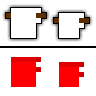# Result of Pixel Shader Invisible on Some Android Devices

I have recently started using pixel shaders to add some cool effects to my game. I recently added a “cloth” in game that would replace parts of player’s characters with a 10x10 repeating texture. It works perfectly for iOS and most Android devices, but some Android devices don’t draw anything. Does anyone have any insight into why this is?

## Here are some screenshots of the problem

Problematic Device:

Working DeviceThe shader uses the R and G values of the mask to determine how to overlay the texture
`

``````sampler s0;
float2 posInTexture;
float2 texSize;
bool hasTex1;
bool hasTex2;
float2 textilePos1;
float2 textilePos2;
float alpha;

texture textile;
sampler textile_sampler = sampler_state
{
Texture = <textile>;
MinFilter = Point;
MagFilter = Point;
MipFilter = Point;
};

sampler mask_sampler = sampler_state
{
MinFilter = Point;
MagFilter = Point;
MipFilter = Point;
};

float mod(int a, int b)
{
return a - (b * floor(a / b));
}

float4 TextileColor(int pX, int pY, sampler text, float2 offset)
{
return tex2D(text, float2(offset.x + mod(pX, 10) / 50, offset.y + mod(pY, 10) / 50));
}

float4 PixelShaderFunction(float2 coords: TEXCOORD0) : COLOR0
{
float4 color = tex2D(mask_sampler, coords);
float4 normColor = tex2D(s0, coords);
float2 localPos = float2((coords.x - posInTexture.x) / texSize.x, (coords.y - posInTexture.y) / texSize.y);
float4 texColor1 = float4(TextileColor(localPos.x * 48, localPos.y * 48, textile_sampler, textilePos1).rgb * color.r, 1);
float4 texColor2 = float4(TextileColor(localPos.x * 48, localPos.y * 48, textile_sampler, textilePos2).rgb * color.g, 1);

if (hasTex1 && color.r > 0) {
return texColor1 * alpha;
}
if (hasTex2 && color.g > 0) {
return texColor2 * alpha;
}
return normColor * alpha;
}

technique Technique1
{
pass Pass1
{
}
}
``````

`

Any help would be greatly appreciated! Thanks

For your pixel shader function, make sure the input parameters match the output parameters of the vertex shader (the SpriteEffect shader in this case). I’ve seen issues because of these not matching before. The outputs are `SV_POSITION, COLOR, TEXCOORD` in that order. I don’t think this causes issues for glsl though.

Secondly some devices might require that your texture dimensions are powers of two. The Pipeline Tool has an option to pad textures for you (ResizeToPowerOfTwo or something like that). Enabling that makes things more portable and hopefully fixes your rendering issue.

Took a while to get our users with problematic devices to test it. They still report the objects are invisible. Anything else that could cause this? It only happens on some Android devices.

Same issue here, on some devices works as expected, on some devices I have invisible graphics. Here is my simple pixel shader

``````int Columns;
int Rows;
float ShiftX;
float ShiftY;

static const float ALPHA_FACTORY = 0.98;

{
float4 position : SV_POSITION;
float4 color : COLOR;
float2 texCoords : TEXCOORD;
};

sampler TextureSampler;

{
const float4 originalColor = tex2D(TextureSampler, input.texCoords);
if(originalColor.a != 0)
{
return originalColor * ALPHA_FACTORY;
}

float calculatedShiftX = ShiftX / (float)Columns;
float calculatedShiftY = ShiftY / (float)Rows;

const float singleFrameWidthProportion = 1 / (float)(Columns);
const float singleFrameHeightProportion = 1 / (float)(Rows);

float fmodX = fmod(input.texCoords.x, singleFrameWidthProportion);
if(fmodX < calculatedShiftX || fmodX + calculatedShiftX > singleFrameWidthProportion)calculatedShiftX = fmodX;

float fmodY = fmod(input.texCoords.y, singleFrameHeightProportion);
if(fmodY < calculatedShiftY || fmodY + calculatedShiftY > singleFrameHeightProportion) calculatedShiftY = fmodY;

float4 newColor = originalColor;
newColor += tex2D(TextureSampler, input.texCoords.xy + float2(calculatedShiftX, calculatedShiftY));
newColor += tex2D(TextureSampler, input.texCoords.xy - float2(calculatedShiftX, calculatedShiftY));
newColor = newColor / 3;

return newColor;
}

// WindowsPhone8 requires SM 4_0_level_9_1 or higher
// Android requires SM 3_0
#ifdef SM4
#define TECHNIQUE(name, vsname, psname ) \
technique Technique1
{
pass Pass1
{
}
}

#else
#define TECHNIQUE(name, vsname, psname ) \
technique Technique1
{
pass Pass1
{
}
}
#endif``````

Any clues how to fix this issue ? As you can see, I use shader model 3.0 for Android.

I am also still getting this issue, it even presents on new devices such as the Galaxy S7, but not on some older devices. Any help would be appreciated.

Have you found solution ? Any clue ? I got it to work on some devices when changed this line:

``const float4 originalColor = tex2D(TextureSampler, input.texCoords);``

to

``const float4 originalColor = tex2D(TextureSampler, input.texCoords) * input.color;``

But still I have blank texture on Samsung Galaxy S8 …